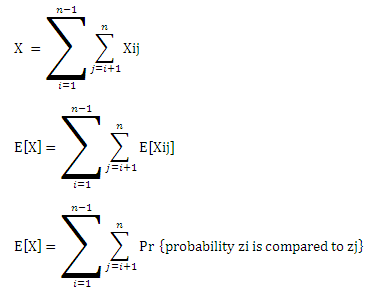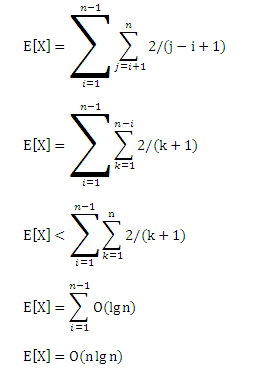# How to randomize Quick Sort and why? Implementation and Analysis of randomized Quick Sort

 Category: Data Structure And Algorithms Tags:Randomized Quick Sort Code Files

## Introduction

In our last article we analyzed quick sort which average case running time is O(n log n). We had some conclusion over worst case and worst case occurs when input is already sorted and if input is random then worst case never occurs, but what If input is not random and somehow algorithm gets input as sorted array?

We will learn solution of above problem in this article but first you must read our last article carefully on Quick Sort because I'm going to use same code with one additional method.

## Explanation

Randomized version of quick sort is nothing but a simple change to get escaped by worst case occurrence. Let's suppose we are having an input array containing 10 numbers from 1 to 10 in sorted manner, If you have read out the last article we can see the worst case would occur in first iteration of for-loop where 10 (if last element of array is pivot) will be the pivot which is already on it's final position so the partitions will be 9(1-9) and 0 elements. then in second iteration, 9 will be the pivot and algorithm will divide input in 8(1-8) and 0 elements and so on.

Now the problem is if we always choose the pivot as last element the algorithm is going to suffer with worst case whenever input is sorted. To solve this problem we have to randomize the pivot selection.

Now think about the scenario we were discussing where input is 10 elements(1-10 array), if our pivot selection was randomized then we would have not ended up pivot element always as last element and then our partitioning wouldn't have been in (n-1) and 0. If we could have selected pivot in random order then there would be mixed scenes of average, worst and best cases instead of having always a worst case.

Making pivot selection randomized would save us from worst case running time and our algorithm would run always on average case which is O(n log n) and that would be still faster then merge/heap sort in real-time scenario because of no unnecessary movements.

## Implementation

We are gonna create only one additional method(RandomizedPartition) in our class(Quick) which implementation can be found in last article.

```public int RandomizedPartition(int p, int r)
{
int random = new Random().Next(p,r);
Swap(random, r);   //making last element is random
return Partition(p,r);
}```

And in our method called QuickSort we call RandomizedPartition instead of Partition, just like that:

```public void QuickSort(int p, int r)
{
if (p < r)
{
int q = RandomizedPartition(p, r);
QuickSort(p, q-1);
QuickSort(q + 1, r);
}
}```

So above we just added a new method called RandomizedPartition and there we generated a random number between start and end index to get a random number and then called Swap to swap our actual pivot element with this random element and then we called old method Partition. So by doing this even if we always choose a last element as pivot actually isn't last, it is random from same set. And from QuickSort we will call RandomizedPartition instead of Partition.

## Analysis

To analyze the algorithm on running time we will use probabilistic analysis because algorithm is random now.

As we know the method QuickSort gives n calls to the method Partition in worst case and log n in best case. We take it as n calls and in method Partition at line 4(if (A[j] <= pivot) check in last article for this code line) where we compare the elements with pivot we says the comparison time is X so the total running time is O(n + X).

Now we have to get value of X to calculate the running time of algorithm, to do so we have to understand when the algorithm compares two elements and when does not. Suppose we have a set of elements Zij = { zi, zi+1, ..., zj } where zi is the ith smallest element.

When does algorithm compare zi and zj? We know that the elements are compared at most once because algorithm compares the elements to pivot and once pivot finalize its position it never gets used or compared to any other element. We are having an indicator I to present the element i compared to j.

Xij = I {zi is compared to zj}

We know an element will not get compared to itself so there is no chance of having z2 compared to z2 (means Z22 will not be a possible pair) so we just can write the value of X like:Above we have the Pr which shows the probability when zi is compared to zj and because one element does not get compared to itself we have the summation i from 0 to n-1 and j is i+1 to n. which come up with all possible sets but not where one gets compared to itself.

To get the probability value Pr we must think about when the 2 elements do not get compared and for that we think about the set of 9 numbers {1,2,3,4,5,6,7,8,9}. If we give this input to the algorithm because pivot selection is randomized now we suppose pivot is 7 so after running Partition method we will be having two sets {1,2,3,3,5,6} and {8,9} and then again Partition will run on two different sets independently so the set-1's (which contains 1-6) any element will never be compared to set-2's (which contains 8-9) elements.

In general if pivot is x (zi < x < zj) then zi will never be compared to zj on condition of zi < x < zj but the same set's element will be compared, like 6 will be compared to any other(1-5) but not with (8-9).

So we can say zi and zj will be compared only if any one of them is chosen as pivot in set {zi, .. zj} .So

Pr {zi is compared to zj} = Pr {zi or zj is chosen as pivot}

= Pr {zi is chosen as pivot} + Pr {zj is chosen as pivot}

= [1 / (j - i + 1)] + [1 / (j - i + 1)]  (total elements are j-i+1)

= 2 / (j - i + 1)

Substituting this value of Pr in above equationSo X is n lg n and the running time of randomized quick sort will be O( n + n lg n) which is O(n log n).

 Like0 PeopleNikhil Joshi Ceo & Founder at Dotnetlovers Atricles: 136 Questions: 12 Given Best Solutions: 12 *

Login via:x